# Is Mean Temp in 2 phase Heat Exchangers Higher Than Logarithmic Mean?

• B
Devin-M
Is Mean Temp in 2 phase Heat Exchangers Higher Than Logarithmic Mean?

I am looking at this paper:

https://docs.lib.purdue.edu/cgi/viewcontent.cgi?article=1314&context=iracc

Having a bit of trouble understanding all of it, but my basic question is just:

If I have a heat pump where the hot side heat exchanger has a gas-liquid phase transition, can the average temperature of the heat exchanger ever be higher than its logarithmic mean temperature?

If we need specific temperatures, suppose the inlet of the heat exchanger is 1000C gas and the outlet is condensed fluid 12C…

Mentor
Is that condensed fluid below saturation? Have you drawn a diagram of the temperature variations of the two streams through the heat exchanger? Are the temperature variations of both streams linear?

Devin-M
Is that condensed fluid below saturation? Have you drawn a diagram of the temperature variations of the two streams through the heat exchanger? Are the temperature variations of both streams linear?
Well I had convinced myself I had found the error in my heat pump + stirling calculation.

First I calculated the Lorenz COP for a given set of heat pump temperatures. Then I used the logarithmic mean temperature of the hot side and cold side to calculate the stirling efficiency. When I multiplied the 2 together it always gives an answer of 1, in other words the stirling isn't generating any excess power.

For example cold in 12C, cold out 4C, hot in 32.1C, hot out 1000C, gives a Lorenz COP of 1.70... and using the logarithmic mean temps of each side, the stirling gets 58.5...% efficiency. Multiply the 2 together and the stirling isn't generating any extra power (1.70... x 0.585... = 1).

But if I use the arithmetic mean temps instead, which are higher than the logarithmic mean, then the stirling gets higher efficiency, and it pushes the combined efficiency over 100%... 1.70... x 0.643 = 1.10...

So my understanding was that in real heat pumps, there are 2 phases in the condenser, and I simply wondered whether the average temp of the condenser could ever be larger than the logarithmic mean.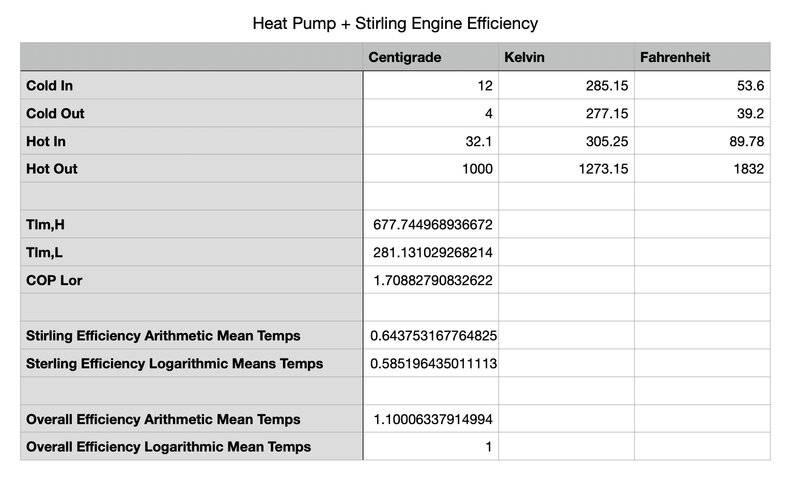Mentor
I looked over that reference you included, and, in my judgment, it is pretty solid. Everything they said makes sense to me.

Devin-M
Is that condensed fluid below saturation? Have you drawn a diagram of the temperature variations of the two streams through the heat exchanger? Are the temperature variations of both streams linear?
Suppose I choose CO2 as a heat pump coolant and in both the cold & hot sides of the heat pump, I want the coolant fluctuating between liquid and gas. The heat source is 275K ocean water.

If I use cold in 275K, cold out 225K, hot in 275K and hot out 400K, I calculate a Lorenz COP of 3.95... and if I use the logarithmic mean of the heat exchangers to calculate the stirling efficiency I get 0.25...

If I multiply those together the answer is exactly 1.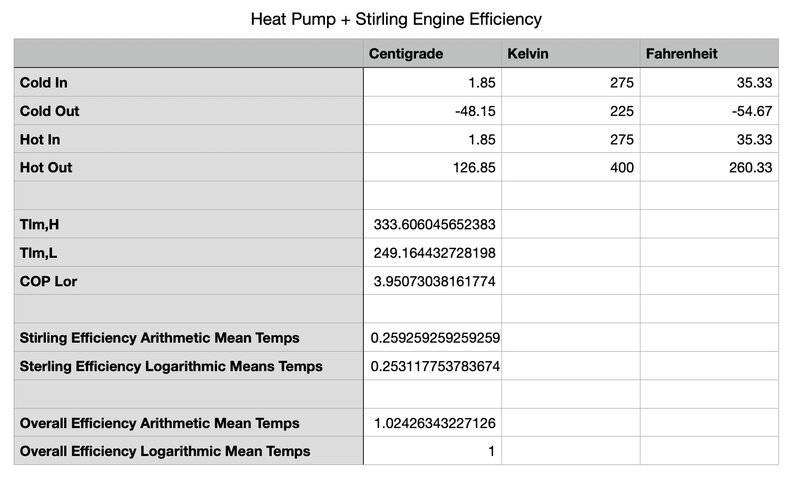CO2 Phase Diagram:...but using the logarithmic mean temp to calculate the stirling efficiency assumes no phase change in the heat exchangers, and in this case, I want liquid and gas in both heat exchangers.

Can you tell me what variables I need to consider and which equations from the paper I should focus on if I want to calculate the average heat of both heat exchangers to calculate the efficiency of the stirling, considering there is a phase change between liquid and gas in both heat exchangers?

Mentor
I am a little confused. Let’s just focus on one heat exchanger. What are the inlet and outlet temperatures on the hot side and cold side, and what are they hot side and cold side fluids. Also what are the flow rates?

Devin-M
I am a little confused. Let’s just focus on one heat exchanger. What are the inlet and outlet temperatures on the hot side and cold side, and what are they hot side and cold side fluids. Also what are the flow rates?
Suppose it's the hot side heat exchanger of the heat pump, the fluid circulating in the heat pump is CO2, at sufficient pressure where the peak temp of the hot side is 400K as a gas and the low temp is 275K as a liquid. Is the average temp higher than the logarithmic mean temp on account of the phase change? Latent heat in addition to sensible heat?

Mentor
Suppose it's the hot side heat exchanger of the heat pump, the fluid circulating in the heat pump is CO2, at sufficient pressure where the peak temp of the hot side is 400K as a gas and the low temp is 275K as a liquid. Is the average temp higher than the logarithmic mean temp on account of the phase change? Latent heat in addition to sensible heat?
Can you please provide a schematic diagram of the system?

Devin-M
Can you please provide a schematic diagram of the system?

To calculate the Lorenz COP, I used equations 2,3 & 4 at:

https://backend.orbit.dtu.dk/ws/portalfiles/portal/149827036/Contribution_1380_final.pdf

To calculate the ideal stirling efficiency, I used equation 1 with the logarithmic mean temps of the hot and cold heat exchangers of the heat pump (but I have my doubts the logarithmic mean temp is actually the average temperature since there is a phase change in the heat exchangers).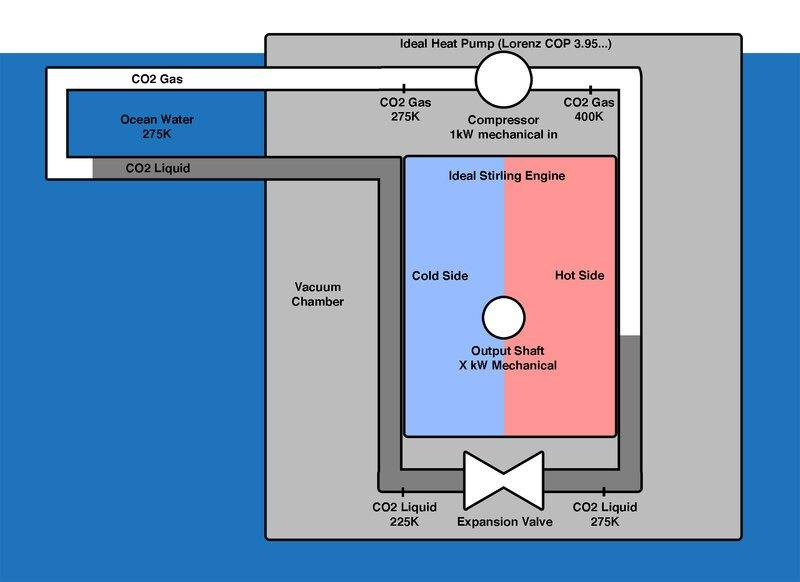Mentor
What is the CO2 pressure when its temperature goes from 400 K to 275 K?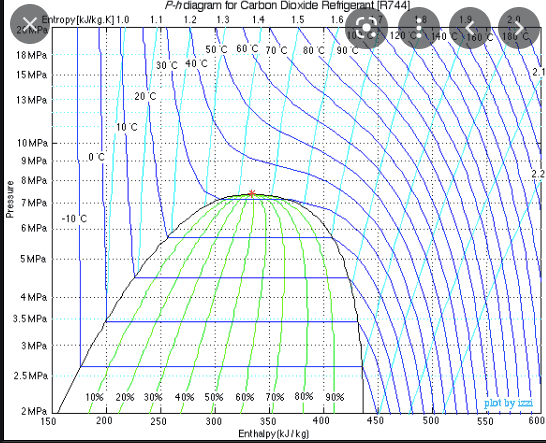Last edited:
Devin-M
I hadn’t considered it, but what if I choose 7.3MPa since it’s right below the critical pressure?

Mentor
I hadn’t considered it, but what if I choose 7.3MPa since it’s right below the critical pressure?
Maybe it’s above?

Devin-M

Mentor
In any event, assuming (temporarily) that the CO2 pressure is approximately constant in the heat exchanger, we have $$\dot{m}\frac{dH}{dA}=-U(T-T_h)$$where ##\dot{m}## is the mass flow rate, H(T) is the enthalpy of the stream (even including liquid) at temperature T, A is the cumulative heat transfer area, U is the overall heat transfer coefficient, and Th is the temperature of the Stirling Engine working fluid (assumed constant) on the hot side. This can be integrated as $$\int_{H_{in}}^{H_{out}}{\frac{dH}{(T-T_h)}}=-\frac{UA}{\dot{m}}$$The only way this is going to lead to the LMTD is if ##dH/dT=C_p## is constant.

Devin-M
I have to admit I’m stepping a little past my area of knowledge here. Is it clear to you whether the hot side avg temp will be equal to, less than or greater than the logarithmic mean temp of the hot side?

Mentor
I have to admit I’m stepping a little past my area of knowledge here. Is it clear to you whether the hot side avg temp will be equal to, less than or greater than the logarithmic mean temp of the hot side?
From the graph in post #10, at 5 MPa, make a graph of T as ordinate versus H as abscissa, running from 2 C to 127 C. Let's see what it looks like.

Devin-M
like this? edit: (fixed)...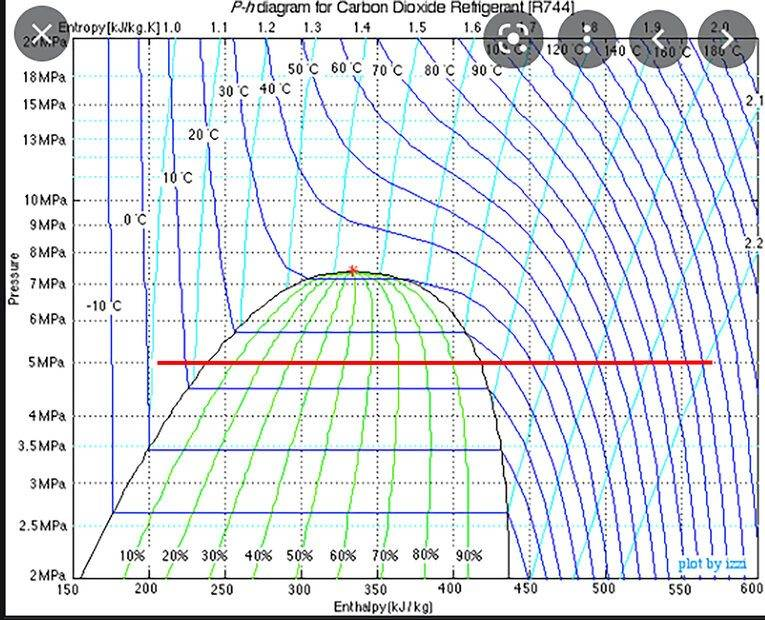Mentor
like this? edit: (fixed)...

View attachment 286283
T as ordinate vs H as abscissa for the interval spanned by the red line.

Devin-M
Here I think I fixed it...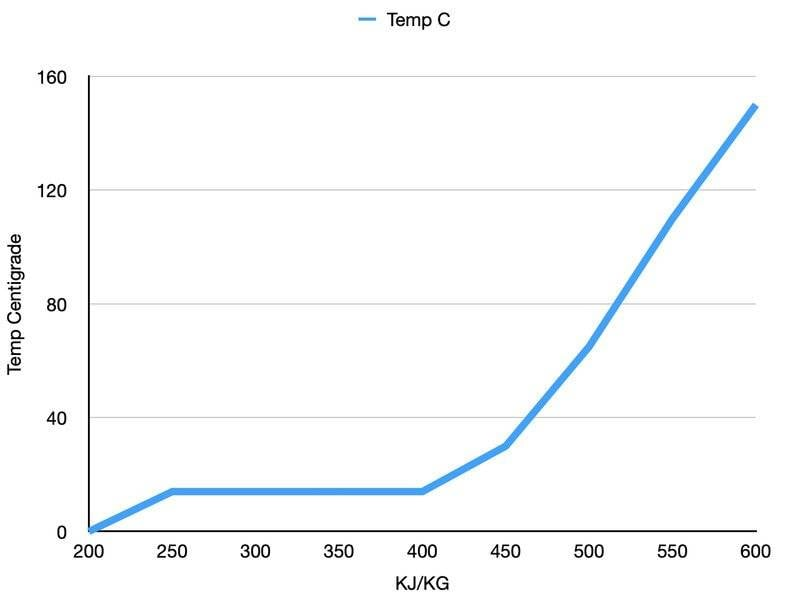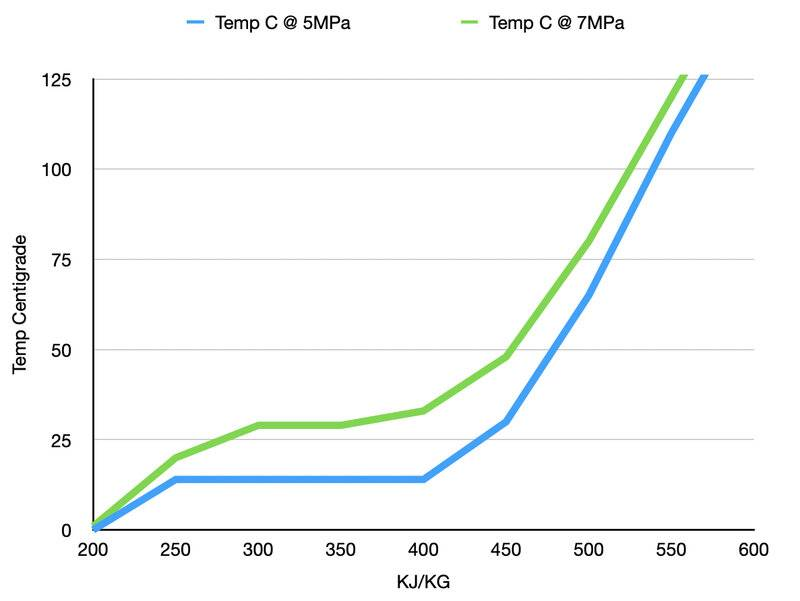Last edited:
Mentor
Here I think I fixed it...

View attachment 286288

View attachment 286289
Excellent. The next step is to draw a straight line between the two end points of each of these variations. This is how the temperature would have to be varying with enthalpy if the LMTD were going to be the correct one to use. Let's see the comparison.

Devin-M
Excellent. The next step is to draw a straight line between the two end points of each of these variations. This is how the temperature would have to be varying with enthalpy if the LMTD were going to be the correct one to use. Let's see the comparison.

I added one more dataset @ 2.5MPa that doesn't phase change in the heat exchanger & stays as gas from 127C to 2C. Compared to the not phase changing 2.5MPa option, the 5MPa and 7MPa options seem to release much more energy per kg for the same temperature change. The 2.5MPa option releases about 120KJ/KG from ~127C to ~2C whereas the 5MPa and 7MPa options release about ~350KJ/KG for the same temperature change or about 2.9x as much energy released at 5MPa and 7MPa for the same temperature change.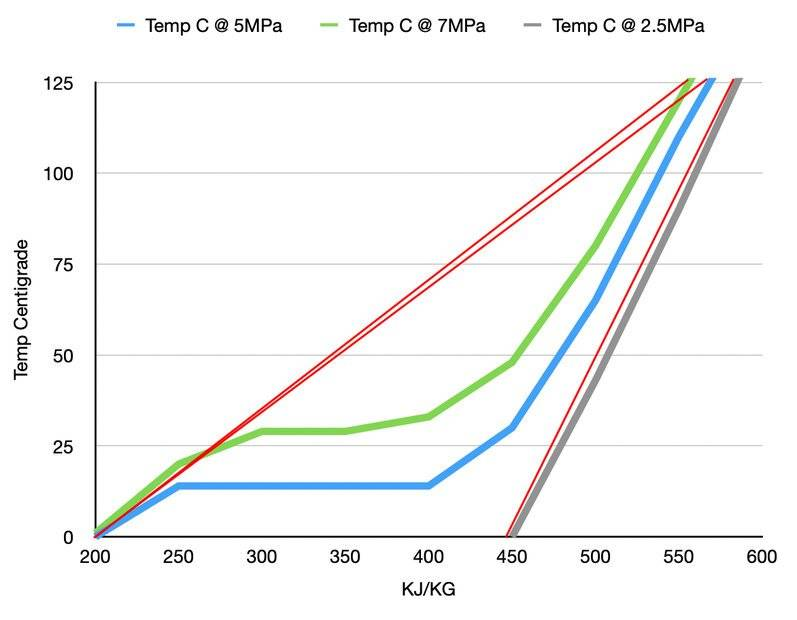Mentor
I added one more dataset @ 2.5MPa that doesn't phase change in the heat exchanger & stays as gas from 127C to 2C. Compared to the not phase changing 2.5MPa option, the 5MPa and 7MPa options seem to release much more energy per kg for the same temperature change. The 2.5MPa option releases about 120KJ/KG from ~127C to ~2C whereas the 5MPa and 7MPa options release about ~350KJ/KG for the same temperature change or about 2.9x as much energy released at 5MPa and 7MPa for the same temperature change.

View attachment 286311
Very nice. You can see that the average temperatures of the CO2 during the heat exchange at 5 and 7 MPa are much lower than implied by the average temperature variation for the straight lines (which would be the variations leading to a log mean temperature difference). Therefore, the correct "effective average" temperature difference to use in the equation ##\dot{m}(H_{out}-H_{in})=-UA\Delta T## is going to be less than the LMTD. If you want to quantify this to compare the correct effective average temperature difference with the LMTD, you can calculate the effective average temperature difference from $$\Delta T=\frac{(H_{out}-H_{in})}{\int_{H_{in}}^{H_{out}}{\frac{dH}{(T-T_h)}}}$$

In the case of the 2.5 MPa (where the CO2 stays a gas), the LMTD would come very close to matching the effective average temperature difference. So the LMTD would be OK for this case.

Devin-M
In the case of the 2.5 MPa (where the CO2 stays a gas), the LMTD would come very close to matching the effective average temperature difference. So the LMTD would be OK for this case.
This part makes sense to me.

You can see that the average temperatures of the CO2 during the heat exchange at 5 and 7 MPa are much lower than implied by the average temperature variation for the straight lines (which would be the variations leading to a log mean temperature difference). Therefore, the correct "effective average" temperature difference to use in the equation m˙(Hout−Hin)=−UAΔT is going to be less than the LMTD.
I'm still confused by this part. Compared to the 2.5MPa case, in, for example, the 7MPa case, for the same amount of KJ/KG released from the C02, say when the enthalpy is down to 350KJ/KG, the temperature is higher in the 7MPA case than the 2.5MPA case. Wouldn't that mean the average temperature is higher than the logarithmic mean, not less?

Mentor
This part makes sense to me.

I'm still confused by this part. Compared to the 2.5MPa case, in, for example, the 7MPa case, for the same amount of KJ/KG released from the C02, say when the enthalpy is down to 350KJ/KG, the temperature is higher in the 7MPA case than the 2.5MPA case. Wouldn't that mean the average temperature is higher than the logarithmic mean, not less?
The temperature is lower during the condensation than if the condensation did not occur. Try integrating that equation I gave numerically to compare the effective average temperature difference with the LMTD.

Devin-M
Would it be accurate to say if phase change occurs in none of the 3 options... at 7MPa, the temp drops from 125C to 50C, and at 5MPa, the temp drops from 125C to 30C, and the 2.5MPa case, the temp drops from 125C to 0C, none of these should have left the gas phase during these temperature swings, and Temp vs KJ/KG line for all of those approximates a straight line. But in the 7MPa and 5MPa cases, if we continue to decrease the temperature, the temperature stays higher for longer for the same amount of energy extracted than would be the case if the logarithmic trend continued, would they not?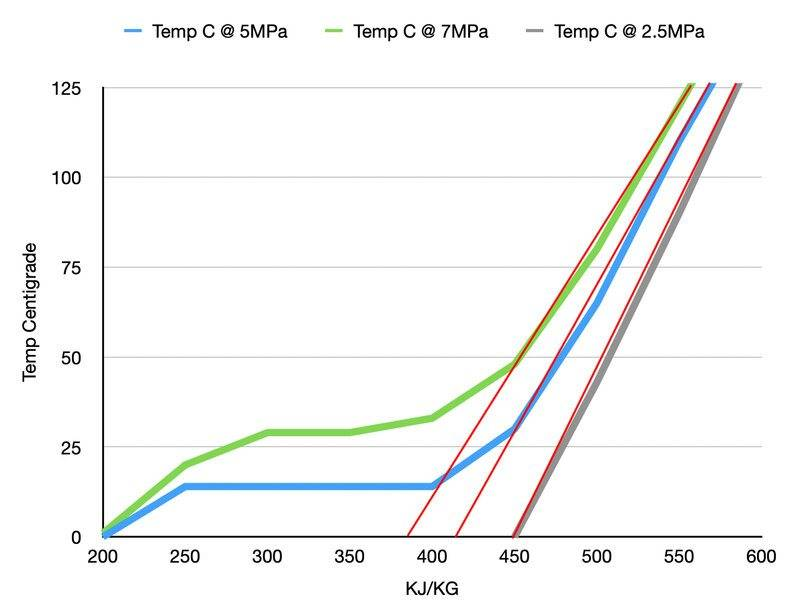Mentor
Would it be accurate to say if phase change occurs in none of the 3 options... at 7MPa, the temp drops from 125C to 50C, and at 5MPa, the temp drops from 125C to 30C, and the 2.5MPa case, the temp drops from 125C to 0C, none of these should have left the gas phase during these temperature swings, and Temp vs KJ/KG line for all of those approximates a straight line. But in the 7MPa and 5MPa cases, if we continue to decrease the temperature, the temperature stays higher for longer for the same amount of energy extracted than would be the case if the logarithmic trend continued, would they not?

View attachment 286318
No exactly. The LMTD and analyses like the present one are typically used to determine the required heat transfer surface area A to bring about the desired amount of heat transfer. The temperature difference between the CO2 and the hot working fluid provides the driving force for the heat exchange, and the lower this temperature difference is, the more area is required. If insufficient heat transfer surface area is provided, the desired amount of heat transfer will not occur (assuming that the overall heat transfer coefficient U is constant). If the cases at 5 MPa and 7 MPa are being designed using the LMTD to determine the heat transfer area, because the LMTD in these cases overestimates the heat transfer driving force, the required heat transfer area will be incorrectly underestimated. A system designed on this basis would not transfer sufficient heat to the working fluid.

Devin-M
No exactly. The LMTD and analyses like the present one are typically used to determine the required heat transfer surface area A to bring about the desired amount of heat transfer. The temperature difference between the CO2 and the hot working fluid provides the driving force for the heat exchange, and the lower this temperature difference is, the more area is required. If insufficient heat transfer surface area is provided, the desired amount of heat transfer will not occur (assuming that the overall heat transfer coefficient U is constant). If the cases at 5 MPa and 7 MPa are being designed using the LMTD to determine the heat transfer area, because the LMTD in these cases overestimates the heat transfer driving force, the required heat transfer area will be incorrectly underestimated. A system designed on this basis would not transfer sufficient heat to the working fluid.

I'm now very confused. Is the below pictogram wrong?

The bottom picture (phase change gas-> liquid) shows a higher average temperature when it reaches equilibrium than the top picture (no phase change). Both have the same starting temperatures. Wouldn't the 7MPa option be more like the bottom picture (higher average temp at equilibrium with the same starting temps on account of phase change latent heat), and the 2.5MPa option more like the top picture (lower average temp at equilibrium with the same starting temps on account of no phase change latent heat)

https://www.speakev.com/cdn-cgi/image/format=auto,onerror=redirect,width=1920,height=1920,fit=scale-down/https://www.speakev.com/attachments/451c3ceb-b873-4f0c-a081-7d7b07705c33-png.147239/

https://www.swepusa.com/refrigerant-handbook/1.-basic-heat-transfer/as5/

Mentor
I'm now very confused. Is the below pictogram wrong?

The bottom picture (phase change gas-> liquid) shows a higher average temperature when it reaches equilibrium than the top picture (no phase change). Both have the same starting temperatures. Wouldn't the 7MPa option be more like the bottom picture (higher average temp at equilibrium with the same starting temps on account of phase change latent heat), and the 2.5MPa option more like the top picture (lower average temp at equilibrium with the same starting temps on account of no phase change latent heat)

https://www.speakev.com/cdn-cgi/image/format=auto,onerror=redirect,width=1920,height=1920,fit=scale-down/https://www.speakev.com/attachments/451c3ceb-b873-4f0c-a081-7d7b07705c33-png.147239/

https://www.swepusa.com/refrigerant-handbook/1.-basic-heat-transfer/as5/
We are not comparing the different pressure cases with one another. We are comparing each of them with the hypothetical temperature vs enthalpy relationship that would have to exist for the CO2 in order for the LMTD to apply. In all three cases, the hypothetical (leading to the LMTD) average temperature of the CO2 profile for heat transfer driving force would be about 60 C, and this would also be the effective average temperature of the CO2 for heat transfer in the 2.5 MPa case. The effective average temperature of the CO2 in the actual 7 MPa case and in the 5 MPa case would be substantially lower than 60 C. In the 7 MPa case, from the graph (by eyeball), I"m guessing an effective average temperature of about 50 C and, in the 5 MPa case, a value of about 40 C.

Devin-M
If I have 1 kg of CO2 at 127C at 2.5MPa, and it's -10C outside, and I let it cool to 0C at constant pressure (by convection to the environment), it has lost 140kJ.

If I have 1kg of CO2 at 127C at 7MPa, and it's -10C outside, and I let it naturally cool by convection so that the same 140kJ exits the 1kg at constant pressure, it's still 35C...

...that's 35C warmer than the 2.5MPa CO2 that has lost the same energy to the environment.

Wouldn't that mean the average temperature is higher with the 7MPa CO2 than the 2.5MPa CO2, because after releasing the same energy, the 7MPa C02 is warmer?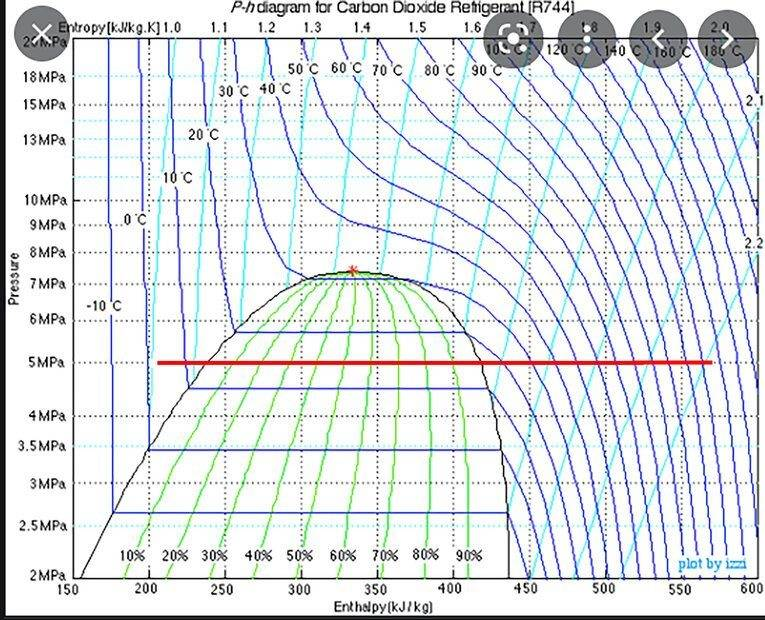Mentor
The comparison should made be for the same temperature change. In that case, for the 7MPa , a larger fraction of the heat is transferred at lower temperatures.

Last edited:
Devin-M
The comparison should made be for the same temperature change.
ok so:

1kg, 7MPa, 127C to 0C goes from 560kJ/kg to 200kJ/kg at constant pressure so loses 360kJ to the -10C environment.

1kg, 2.5MPa, 127C to 0C goes from 590kJ/kg to 450kJ/kg at constant pressure so loses 140kJ to the -10C environment.

Since the environment acquires 220kJ more with the 7MPa than the 2.5MPa for the same temperature change, doesn't that make the 7MPa, warmer on average, and wouldn't it mean the 2.5MPa loses it's temperature according to the logarithmic formula since it has no phase change (stays as a gas) while the 7MPa converts from a gas to a liquid?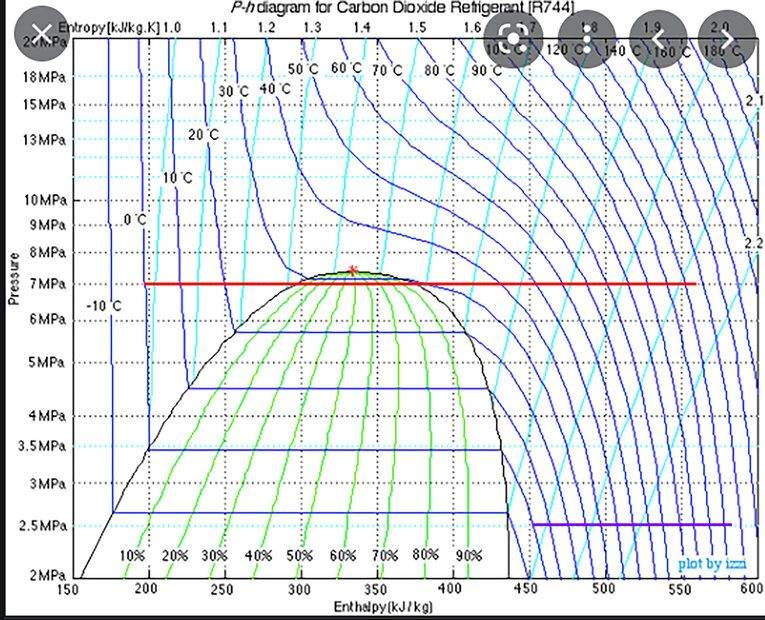Mentor
The 2.5 would definitely follow the log formula. The 7.5 would transfer more heat with a lower average temperature difference (relative to the -10 C), and would thus require moch more heat transfer area A.

Devin-M
If the -10C environment is sealed and insulated, and the 2.5MPa 1kg CO2 drops from 127C to 0C (remaining a gas), and at that point the experiment is reset and repeated with the 7MPa 1kg from 127C to 0C, by the time the environment has warmed to the same temperature as the end of the first experiment, the 7MPa 1kg is still 35C. Wouldn't a thermometer measure the cube 35C warmer at that point in the experiment? If the cube is measured warmer, won't heat transfer still be occurring at a higher rate than the first experiment? If that's all true how could could the average temperature of the 7MPa 1kg as tabulated at any point in the experiment be lower?

Mentor
I’m obviously having a hard time explaining what I mean. I think I’ll stop for now and give others a chance to respond.

Devin-M
I really do appreciate the help you've given and I feel my understanding has advanced thanks to you but still not fully grasping it.